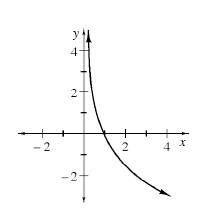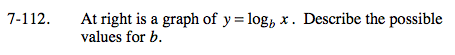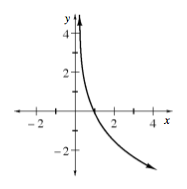Home > A2C > Chapter 7 > Lesson 7.2.2 > Problem7-112

7-112.

Below is a graph of y = logb x. Describe the possible values for b. 7-112 HW eTool (CPM). Homework Help ✎Think of the graph of log(x). How is this graph different?
How would that affect the equation?
What is the maximum that b could be? What is the minimum?Use the eTool below to see how different values of b change the graph.
Click the link at right for the full version of the eTool: A2C 7-112 HW eTool